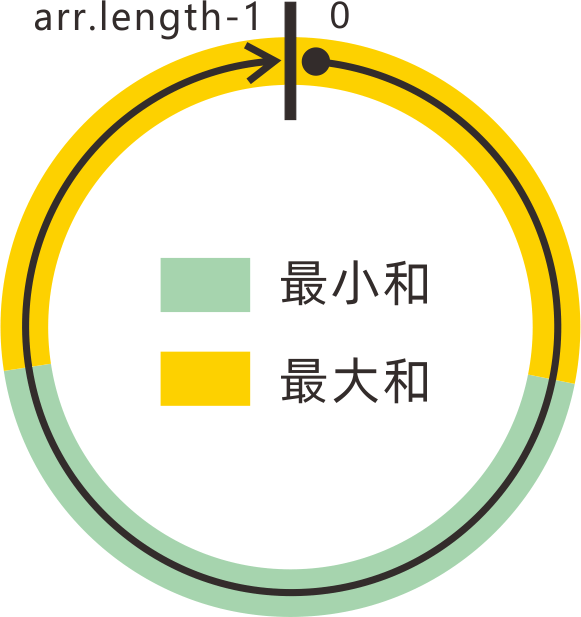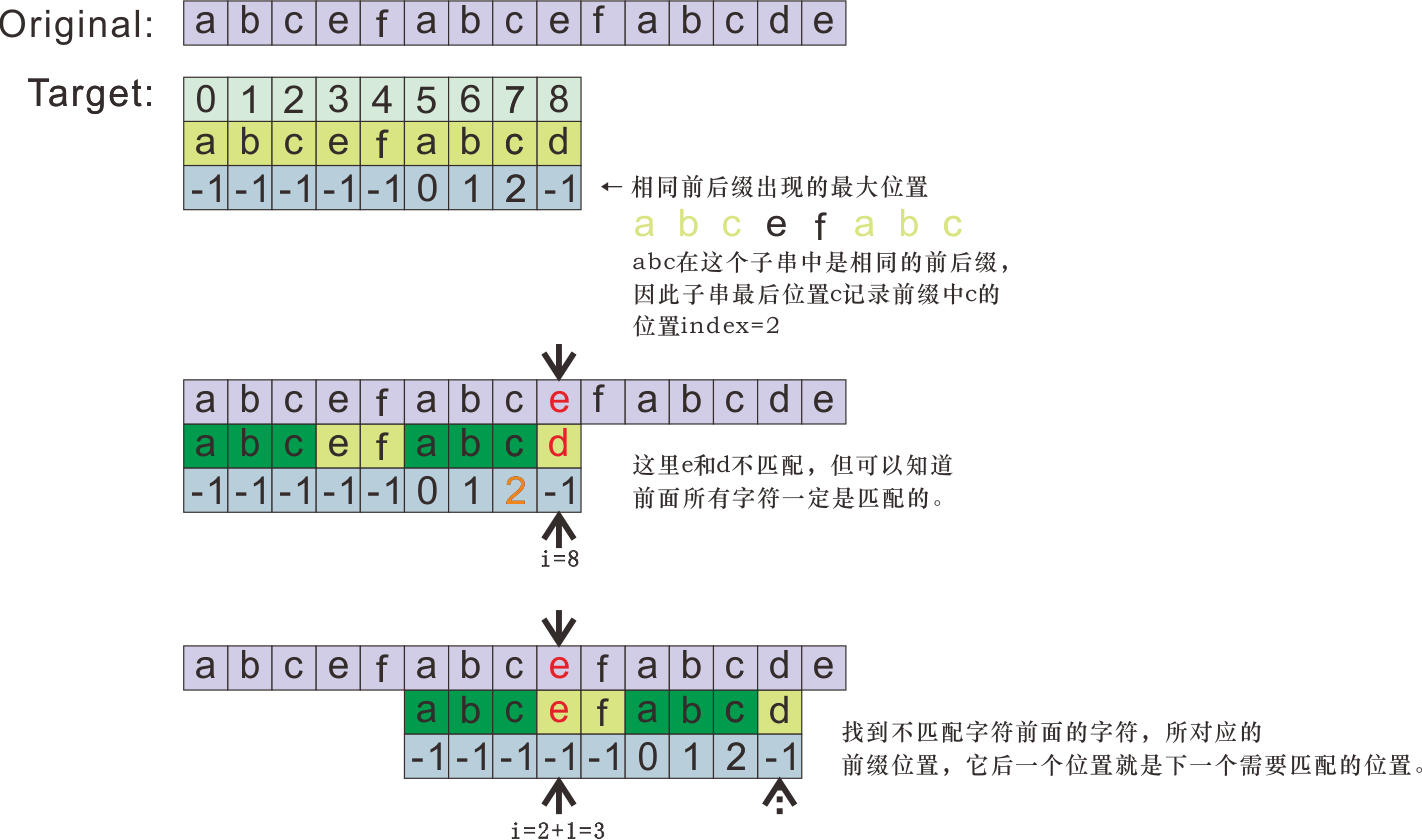# 经典算法

Posted by Mars at

## 1.1 计算连续子数组最大和、最小和

【1.求子数组最大和】

（子数组：在原数组中必须是连续的，长度最小为1。单个元素构成的数组和数组A本身也是A的子数组。）

• 最终求得的子数组SubA一定以数组A中某一个元素Ai作为结尾；
• SubA在原数组A中是连续的。

① 继续使用Ai结尾的最大子数组，SubAi+1的加和可以表示为K+Ai+Ai+1，也就是 Sum（Ai+1）= Sum（Ai）+Ai+1

② 不继续沿用之前Ai的最大子数组，而是自己单独成为一个子数组，如果之前的子数组SubAi的加和Sum（Ai对它而言是个累赘（为负）。这时Sum（Ai+1） = Ai+1;

1. 初始化 maxSumHere = Amax = A;
2. 对原数组A从左到右进行遍历，计算以每个数组元素位置i为结尾的子数组加和值maxSumHere，计算方法为：Sum（Ai+1）=Math.max(Sum（Ai）+Ai+1, Ai+1)；
3. 当前元素i计算完成后，更新max值。（最终结果为各个元素为结尾位置，而得到的子数组加和集的最大值。）
4. 返回max值即为结果。
``````// Kadane's Algorithm
let lastSubArrayMax = array;
let totalMax = array;
for(let i=1; i<array.length; i++){
// Sum（Ai_+_1）=Math.max(Sum（A_i）+A_i_+_1, A_i_+_1)；
if(lastSubArrayMax + array[i] < array[i]) lastSubArrayMax = array[i];
else lastSubArrayMax = lastSubArrayMax + array[i];

// Refresh the total Max Value.
totalMax = Math.max(lastSubArrayMax, totalMax);
}
}
``````

``````// Kadane's Algorithm
// Max / Min
let curMax = arr; // let curMin = arr
let max = arr;  // let min = arr;
for(let i=1; i<arr.length; i++){
curMax = Math.max(curMax+arr[i], arr[i]);  //  curMin = Math.min(curMin+arr[i], arr[i]);
max = Math.max(max, curMax); // min = Math.min(min, curMin);
}
return max;
}
``````

## 1.2 计算环形数组的连续子数组最大和、最小和

1. 最大子数组包含首尾两个元素（覆盖了首尾连接成环的间隙）；
2. 最大子数组出现在原数组内部（不覆盖首尾连接成环的间隙）。``````var maxSubarraySumCircular = function(nums) {
let sum = nums;
let curMin = nums, curMax = nums;
let min = curMin, max = curMax;
for(let i=1; i<nums.length; i++){
sum += nums[i];
curMax = Math.max(curMax+nums[i], nums[i]);
curMin = Math.min(curMin+nums[i], nums[i]);
max = Math.max(max, curMax);
min = Math.min(min, curMin);
}
// 全部元素为负，返回max而非0.
return max < 0 ? max : Math.max(max, sum-min);
};
``````

## 1.3 计算连续子数组乘积的最大值、最小值

1. 如果下一个元素为正，则包含下一个元素数组的最大乘积`max[i] = max[i-1] * num[i]`，最小乘积`min[i] = min[i-1] * num[i]`;
2. 如果下一个元素为负，则包含下一个元素数组的最大乘积`max[i] = min[i-1] * num[i]`，最小乘积`min[i] = max[i-1] * num[i]`

``````var maxProduct = function(nums) {
let curMax = nums;
let curMin = nums;
let max = curMax, min = curMin;
for(let i=1; i<nums.length; i++){
// 无论情况如何，乘积最大值应该是这三者中的最大者，乘积最小值应该是最小者。
let a1 = curMax*nums[i], a2 = curMin*nums[i], a3 = nums[i];
curMax = Math.max(a1, a2, a3);
curMin = Math.min(a1, a2, a3);
max = Math.max(max, curMax);
min = Math.min(min, curMin);
}
return max;
};
``````

# 2. KMP算法

KMP算法，用于解决查询字符串str1中是否存在子字符串str2的问题。

# 2.1 算法内容``````function knuthMorrisPrattAlgorithm(string, substring) {

function getSuffixPosition(str){
let suffixPosition = new Array(str.length);
suffixPosition.fill(-1);
let j = 0;
for(let i=1; i<str.length; i++){
if(str[i] === str[j])	suffixPosition[i] = j++;
else j=0;
}
return suffixPosition;
}

let suffixPosition = getSuffixPosition(substring);

let i=0, j=0;
while(i < string.length){
if(string[i] === substring[j]){
if(j === substring.length-1) return true;
j++; i++;
}
else {
if(j === 0) i++;
else j = suffixPosition[j-1]+1;
}
}

return false;
}
``````

# 2,2 复杂度分析

m为查询子串长度，n为被查询原字符串长度。

Keywords: Algorithm